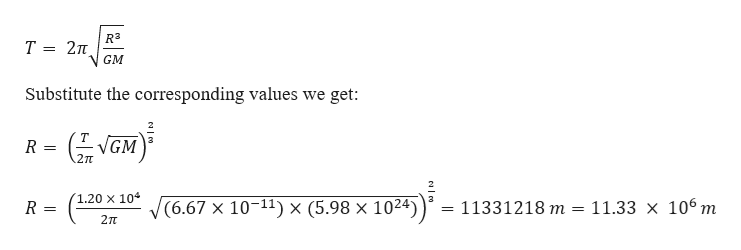# A satellite is in a circular orbit about the earth (ME = 5.98 × 1024 kg). The period of the satellite is 1.20 × 104 s. What is the speed at which the satellite travels?

Question

A satellite is in a circular orbit about the earth (ME = 5.98 × 1024 kg). The period of the satellite is 1.20 × 104 s. What is the speed at which the satellite travels?

check_circle

Step 1

Given information:

Mass of the earth (M) = 5.98 × 1024 kg

Time period of satellite (T) = 1.20 × 104 s

General information:

Universal gravitational constant (G) = 6.67 × 10-11 m3 kg-1s-2

Step 2

We know that the time period of t...help_outlineImage TranscriptioncloseR3 T 2nt GM Substitute the corresponding values we get: VGM 2 R 1.20 x 104 (6.67 x 10-11) x (5.98 x 1024) 11.33 x 106 m 11331218 m R 2TT fullscreen

### Want to see the full answer?

See Solution

#### Want to see this answer and more?

Solutions are written by subject experts who are available 24/7. Questions are typically answered within 1 hour.*

See Solution
*Response times may vary by subject and question.
Tagged in

### Physics